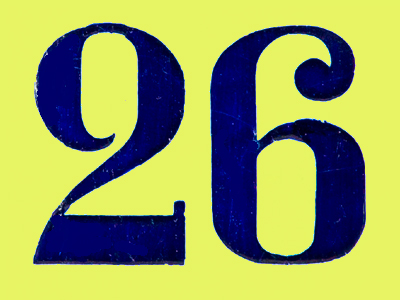26 is the next number of the sequence 5 6 8 11 15 20.

# Number Sequences (Year 6)

In KS2 Maths Year Six, kids are getting really good at number sequences. They can easily see patterns in numbers going up or down, including whole numbers, fractions, and decimals. They know odd and even numbers well and understand what happens when you add, subtract, or multiply them. Now, they're discovering sequences with square, triangle, and minus numbers!

Number sequences are like magic lists of numbers with patterns. For example, triangle numbers go up by 1, then 2, then 3, and so on. Square numbers go up by 3, then 5, then 7, and so on. Spotting these patterns helps you figure out the next numbers in the sequence.

Can you find square and triangle number sequences? Test your skills in this quiz for 10-11 year olds!

1.
Can you find the rule of this sequence? 21, 42, 63, 84
The numbers increase in 11s
The numbers increase in 19s
The numbers increase in 21s
The numbers increase in 25s
This sequence is the 21 times table
2.
Can you find the rule of this sequence? 30, 45, 60, 75, 90
The numbers increase in 11s
The numbers increase in 15s
The numbers increase in 19s
The numbers increase in 21s
Each number (except the first) is 15 more than the one before it
3.
What is the next number of the sequence? 12, 9, 6, 3, 0
-3
3
0.3
-6
The numbers are decreasing in 3s
4.
What is the next number of the sequence? 0.5, 1, 1.5, 2
1
2.5
3
3.5
The numbers are increasing by 0.5 each time
5.
What is the next number of the sequence? 1, 3, 6, 10, 15, 21
26
27
28
30
Number 2 is added, then 3, then 4, then 5 and so on
6.
What do we call the numbers in the sequence in question 5?
Multiples
Square numbers
Prime numbers
Triangular numbers
The sequence of triangular numbers goes up much more quickly than an ordinary sequence. Square numbers go up even more quickly
7.
The difference between two even numbers is what?
Sometimes odd and sometimes even
Always odd
Always even
It always ends in 0
Even - even = even
8.
The product of two even numbers is what?
Sometimes odd and sometimes even
Always odd
Always even
It always ends in 0
A product is the result of multiplying two or more numbers
9.
The product of two odd numbers is what?
Sometimes odd and sometimes even
Always odd
Always even
It always ends in 0
The product of two evens is always even and the product of two odds is always odd
10.
The product of an even number and an odd number is what?
Sometimes odd and sometimes even
Always odd
Always even
It always ends in 0
Even x even = even
Even x odd = even
Odd x even = even
Odd x odd = odd
You can find more about this topic by visiting BBC Bitesize - What is a number sequence?

Author:  Amanda Swift